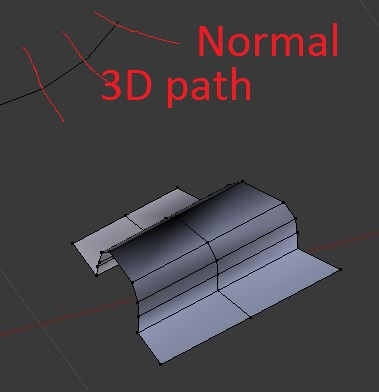# mathutil vector and edges

I got a mesh path line in 3D
and need to rotate some verts normal to the edge along that path

I can calculate the derivative and rotate at 90

but is there something simpler that can be used using mathutil vectors may be?

saw this example but not what I want

``````

import bpy
import bmesh
me = bpy.context.object.data
bm = bmesh.from_edit_mesh(me)
active_edge = None
for elem in reversed(bm.select_history):
if isinstance(elem, bmesh.types.BMEdge):
active_edge = elem
break
else:
raise Exception("No active edge!")
v1, v2 = active_edge.verts
#co1 = v1.co + v1.normal
#co2 = v2.co + v2.normal
n = (v1.normal + v2.normal) * 0.5
co1 = v1.co + n
co2 = v2.co + n
ret = bmesh.ops.duplicate(bm, geom=[active_edge])
new_verts = [elem for elem in ret['geom'] if isinstance(elem, bmesh.types.BMVert)]
# Order???
new_verts.co = co1
new_verts.co = co2
bmesh.update_edit_mesh(me)

``````

need to be at 90 degrees or normal at the end of the edge

thanks

Actually there’s an awesome vector function called “rotate” than can do all this for you.

``````
&gt;&gt;&gt; import math
&gt;&gt;&gt; v = Vector( (0,0,1) )
&gt;&gt;&gt; e = Euler( ( math.radians(90), 0, 0 ) )
&gt;&gt;&gt; v.rotate( e )
&gt;&gt;&gt; v
Vector((0.0, -1.0, -4.371138828673793e-08))

``````

It’s worth noting, though, that the rotate function doesn’t just return a new vector, it actually affects the Vector in question, and will rotate normals, vertices, locations, etc.

If you want to just do a simple calculation, it’s best to first create a copy of the vector in question:

``````v2 = v1.copy()
``````

let say that you have a mesh 3d path = line
you can loop over all verts along the path
then for each edge you can make a vector
then do some calculations to get the normal to that vector

and that is the angle I need to change the rotation of another set of verts being move rotated at same angle then the normal
hopefully it can be done in 3D
I can show dwg for thisthanks

an edge has an infinite number of orthogonal vectors, you need a third coordinate

you make the edge a vector then there is only one normal
like bevel curve along a curve !
the bevel curve is always at 90 degrees or normal to the curve!

happy bl